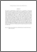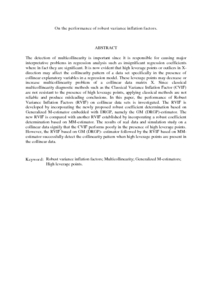# On the performance of robust variance inflation factors.

## Citation

Bagheri, A. and Midi, Habshah (2011) On the performance of robust variance inflation factors. International Journal of Agricultural and Statistical Sciences , 7 (1). pp. 31-45. ISSN 0973-1903

## Abstract

The detection of multicollinearity is important since it is responsible for causing major interpretative problems in regression analysis such as insignificant regression coefficients where in fact they are significant. It is now evident that high leverage points or outliers in X-direction may affect the collinearity pattern of a data set specifically in the presence of collinear explanatory variables in a regression model. These leverage points may decrease or increase multicollinearity problem of a collinear data matrix X. Since classical multicollinearity diagnostic methods such as the Classical Variance Inflation Factor (CVIF) are not resistant to the presence of high leverage points, applying classical methods are not reliable and produce misleading conclusions. In this paper, the performance of Robust Variance Inflation Factors (RVIF) on collinear data sets is investigated. The RVIF is developed by incorporating the newly proposed robust coefficient determination based on Generalized M-estimator embedded with DRGP, namely the GM (DRGP)-estimator. The new RVIF is compared with another RVIF established by incorporating a robust coefficient determination based on MM-estimator. The results of real data and simulation study on a collinear data signify that the CVIF performs poorly in the presence of high leverage points. However, the RVIF based on GM (DRGP)- estimator followed by the RVIF based on MM-estimator successfully detect the collinearity pattern when high leverage points are present in the collinear data.Preview
PDF (Abstract)
On the performance of robust variance inflation factors.pdfView Item